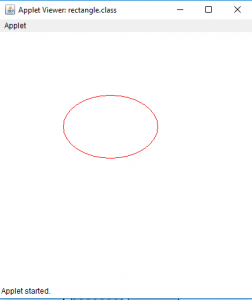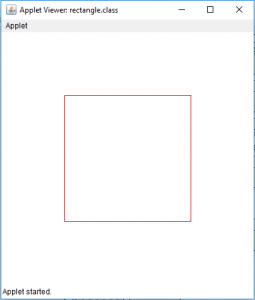Draw a ellipse and a rectangle in Java Applet

• Difficulty Level : Hard
• Last Updated : 06 Jun, 2018

Java applets are application that can be executed in web browsers or applet viewers . We can draw shapes on the Java applet.
In this article we will draw a ellipse on Java applet by two ways . By using the drawOval(int x, int y, int width, int height) or by using mathematical formula (X= A * sin a, Y= B *cos a, where A and B are major and minor axes and a is the angle ) .
Similarly, we will draw a rectangle on Java applet by two ways . By using the drawRect(int x, int y, int width, int height) or by drawing four lines joining the edges .

To draw a ellipse in Java Applet
Examples:
Let us draw a oval with width 150 and height 100

Input : x and y coordinates 100, 100 respectively
Width and height 150 and 100 respectively

Output :To draw a rectangle in Java Applet
Examples:
We will draw a rectangle of height 200 and width 200 and
At a position 100,100 on the applet.

Input : x and y coordinates 100, 100 respectively
Width and height 200 and 200 respectively.

Output :1. Java Program to draw a ellipse using drawOval(int x, int y, int width, int height)

 // java program to draw a ellipse// using drawOval function.import java.awt.*;import javax.swing.*;  public class ellipse extends JApplet {      public void init()    {        // set size        setSize(400, 400);          repaint();    }      // paint the applet    public void paint(Graphics g)    {        // set Color for rectangle        g.setColor(Color.red);          // draw a ellipse        g.drawOval(100, 100, 150, 100);    }}

Output :2. program to draw a ellipse using drawLine function

 // java program to draw a ellipse// using drawLine functionimport java.awt.*;import javax.swing.*;  public class ellipse extends JApplet {      public void init()    {        setSize(300, 300);    }      public void paint(Graphics g)    {        // center of the        int cx, cy;          // center of th ellipse        cx = 150;        cy = 175;          // major and minor axis        double A = 75, B = 50, px = 0, py = 0;          // set color        g.setColor(Color.red);          // draw the ellipse        for (int i = 0; i <= 360; i++) {            double x, y;            x = A * Math.sin(Math.toRadians(i));            y = B * Math.cos(Math.toRadians(i));              if (i != 0) {                // draw a line joining previous and new point .                g.drawLine((int)px + cx, (int)py + cy,                                (int)x + cx, (int)y + cy);            }              // store the previous points            px = x;            py = y;        }    }}

Output :Now we will see how to draw a rectangle in a Java Applet

We can draw rectangle in java applet by two ways.
1. Draw a rectangle using drawRect(int x, int y, int width, int height)

 // Java Program to  Draw a rectangle// using drawRect(int x, int y, int width, int height)import java.awt.*;import javax.swing.*;  public class rectangle extends JApplet {      public void init()    {        // set size        setSize(400, 400);          repaint();    }      // paint the applet    public void paint(Graphics g)    {        // set Color for rectangle        g.setColor(Color.red);          // draw a rectangle        g.drawRect(100, 100, 200, 200);    }}

Output:2. Draw a rectangle using drawLine(int x, int y, int x1, int y1)

 // Java Program  Draw a rectangle// using drawLine(int x, int y, int x1, int y1)import java.awt.*;import javax.swing.*;  public class rectangle extends JApplet {      public void init()    {        // set size        setSize(400, 400);          repaint();    }      // paint the applet    public void paint(Graphics g)    {        // set Color for rectangle        g.setColor(Color.red);          // draw a rectangle by drawing four lines        g.drawLine(100, 100, 100, 300);        g.drawLine(100, 300, 300, 300);        g.drawLine(300, 300, 300, 100);        g.drawLine(300, 100, 100, 100);    }}

Output :Note : the following programs might not run in an online compiler please use an offline IDE.

My Personal Notes arrow_drop_up